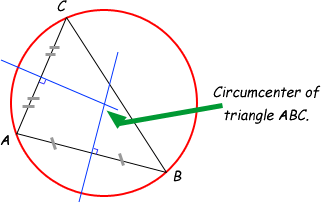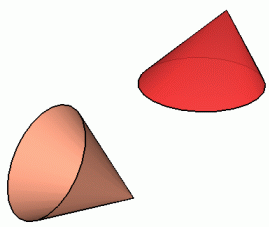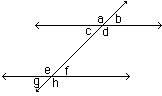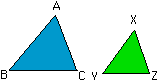Math Central - mathcentral.uregina.caResource Room— glossaries Resource RoomMathematics Glossary - Middle Years
Maxine Stinka, Saskatchewan Education, Curriculum and Instruction

Note: The definitions included here are those that are used in the Saskatchewan Education document "Mathematics 6-9: A Curriculum Guide for the Middle Level".

Various mathematics dictionaries may have different definitions. These definitions are designed to be meaningful to middle level mathematics teachers.

Select the first letter of the term that you wish to look for.
A | B | C | D | E | F | H | I | J | K | L | M | N | O | P | Q | R | S | T | U | V | W | X | Y | Z

C

capacity

• the amount a container holds.

census

• when information is gathered from all people in the population, the activity is called a census. Polls or surveys are a method of collecting data by asking people to give their answers to a set of questions.

central angle of circle

• the angle formed at the centre of a circle by the intersection of two radii.

central angle of a regular polygon

• an angle at the centre of the polygon .

centroid

• the point of intersection of the three medians of a triangle.

• the point around which an object is rotated. (see rotation )

• a segment joining two points on a circle.

circle graph

• a graph of statistical data where a circle is subdivided into regions that represent the percent of the total.

circumcenter of a triangle

• The point which is the center of a circle that includes the vertices of the triangle on its circumference. The circumcenter can be found by looking at the intersection of the perpendicular bisectors of the any two of the sides of the triangle.• the distance around (perimeter of) a circle.

coefficient

• the numerical factor of a term. e.g. The coefficient of -3x2y is -3. The coefficient of a3b4c2 is 1.

commission

• earnings based on the amount of total sales.

commutative property

• the order of numbers in a calculation does not affect the result. e.g. Addition is commutative since a + b = b + a. E.g. 3 + 5 = 5 + 3. Subtraction is not commutative since 8 - 2 and 2 - 8 are not equal.

compatible numbers

• numbers that are easy to manipulate mentally. e.g. 25 X 4, 8 + 2

compensation

• adjusting an estimated answer up or down to more closely approximate the value.

complementary angles

• two angles whose measures total 90o.

composite number

• a whole number (integer) that has more than 2 different factors. e.g. 18 has factors 1, 18, 2, 9, 3, 6 so it is composite.

compound bar graph

• a bar graph that compares two or more quantities simultaneously.

compound event

• the outcome of a probability experiment that involves more than one object. e.g. when you roll two dice and the result is a 5 on one and a 2 on the other, this is a compound event.

compound interest

concave polygon

• a polygon with at least one interior angle with measure greater than 180o.

cone

• the surface or solid formed by rays emanating from a fixed point (vertex) passing through a closed planar curve called the base.
• In common usage, the word cone refers to a right circular cone, which is a cone whose base is a circle and whose vertex is directly above or below the center of the circle.• figures that have the same size and same shape.
• angles

• angles that have the same measure.

triangles

• triangles that are the same size and shape.

convex polygon

• a polygon with each interior angle measuring less than 180o.

• a plane that is divided into four quadrants by drawing a vertical and a horizontal line that intersect at a point called the origin. Used for graphing ordered pairs, the coordinates .
• the ordered pair that names the location of a point on the coordinate plane. The first number in the ordered pair is called the abscissa and the second number is the ordinate.

corresponding angles

• angles that have the same relative positions.
• in parallel lines corresponding angles are congruent.

 pairs of corresponding angles are: a and e b and f c and g d and hin triangles pairs of corresponding angles are: < A and < X < B and < Y < C and < ZCorresponding angles are congruent if the triangles are similar or congruent.

cube root

• a number that when cubed (taken to the power of 3) gives the original number.about math central :: site map :: links :: notre site français* Registered trade mark of Imperial Oil Limited. Used under license.